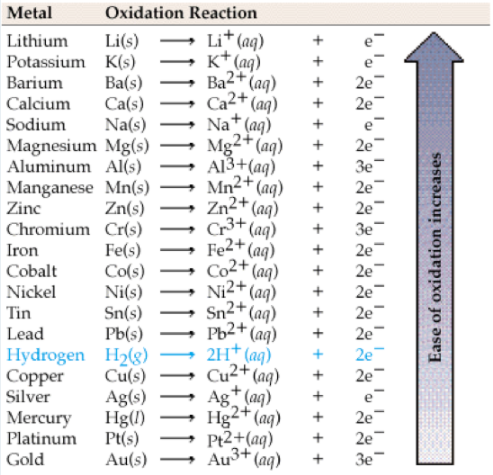# Problem: Write the balanced molecular equation (ME) and net ionic equation (NIE) for the following reaction. Be sure to include the correct states in the final equation and charges for ions in the NIE. CuCl2(aq) + Pb(s) → ME:   NIE:

###### Problem Details

Write the balanced molecular equation (ME) and net ionic equation (NIE) for the following reaction.

Be sure to include the correct states in the final equation and charges for ions in the NIE.

CuCl2(aq) + Pb(s) →

ME:

NIE: